• 雅可比矩阵首先我们求速度，一般指的是机械臂端部的速度。所以对端部位姿求微分，可写为 。其中 为末端沿xyz轴的微分运动， 为末端绕xyz的微分旋转。而我们也已经知道端部位姿矩阵关于关节变量的方程，例如 对其求...
按照我们的初级进阶之路，位置搞定了，下一步该是速度了。速度可以表示为位置的微分形式，所以对机器人的微分运动分析就可以进行速度分析。雅可比矩阵首先我们求速度，一般指的是机械臂端部的速度。所以对端部位姿求微分，可写为。其中为末端沿xyz轴的微分运动，为末端绕xyz的微分旋转。而我们也已经知道端部位姿矩阵关于关节变量的方程，例如对其求时间微分，等式右边对关节变量求导，可得到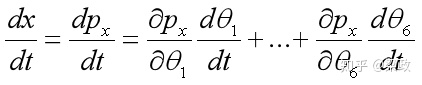去掉dt，可得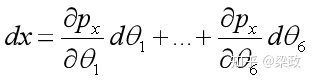那么，用同样的方式计算，可得相同形式，总结为如下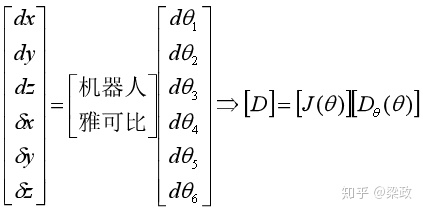其中矩阵为机器人的雅可比矩阵（Jacobian）。它描述了一种关系，可以将转动关节的微分运动表示成末端关节的微分运动。注意，雅可比矩阵不是常数矩阵，他随着关节角变化而变化。像正逆运动学一样，知道了转动关节，也就是电机的角速度，我们就可以求出末端关节的位姿变化速度；另一方面，有了对位姿变化速度的要求，就可以对电机角速度进行设计。这次就不手撕雅可比了，直接上代码吧。假设我们的，看看雅可比矩阵和位姿增量为多少。用rbt的话直接robot.jacob0(theta_d)。L(1)=Link('d',105.03,'a',0,'alpha',pi/2,'standard');
L(2)=Link('d',0,'a',-174.42,'alpha',0,'offset',-pi/2,'standard');
L(3)=Link('d',0,'a',-174.42,'alpha',0,'standard');
L(4)=Link('d',75.66,'a',0,'alpha',pi/2,'offset',-pi/2,'standard');
L(5)=Link('d',80.09,'a',0,'alpha',-pi/2,'standard');
L(6)=Link('d',44.36,'a',0,'alpha',0,'standard');
robot=SerialLink(L(1:6),'name','Gluon_6L3', 'manufacturer','innfos')
theta=deg2rad(15*ones(6,1));
T=robot.fkine(theta).T;
D_theta = deg2rad(0.1*ones(6,1));
J = robot.jacob0(theta)  %Jacob Matrix
D = J*D_theta
结果为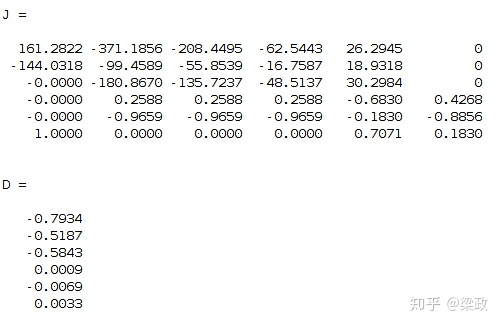这里表示的6自由度位姿的增量。微分变换在得到位姿的增量后，我们还应该求得位姿T矩阵的增量和变换后的位姿T矩阵。按照基础T矩阵坐标变换，位姿矩阵增量应该是根据位姿得坐标变换得来的。我们的位姿增量[D]为微分形式，所以叫微分变换。微分平移，；微分旋转，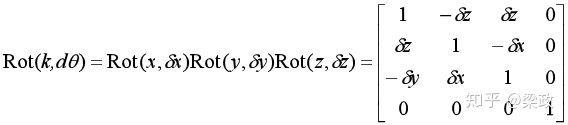因为是微分项，所以求可近似。近似后旋转顺序可忽略，这样旋转可认为是绕k轴旋转d_theta角，再将高阶项省略。由微分旋转可得变换后的矩阵I为单位阵，我们称为微分算子。归纳一下，微分算子为然而，此处的表示的是相对于固定参考坐标系的微分算子。还有其他微分算子，比如相对于当前坐标系的微分算子。这个之后要用到，所以要求一下。相对于固定的左乘，相对于当前的就要右乘。结果应该是一样的，以为描述的相同的变化。所以归纳一下，相对于当前坐标系的微分算子为其中，，，，，。这样我们就可以利用关节转角微分->雅可比矩阵->位姿微分->微分算子->末端微分运动位姿矩阵dT->末端位姿矩阵我们利用matlab求一下% 接上部分
delta = [0   -D(6)  D(5) D(1);
D(6) 0    -D(4) D(2);
-D(5) D(4)  0    D(3);
0    0     0    0]    %微分算子
dT = delta*T
T_new = dT+T结果如下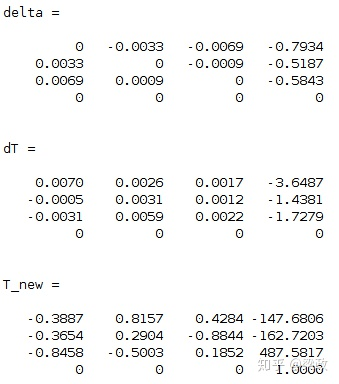展开全文• 因此，这个问题的最终核心在于：如何快速求解一个稀疏大规模的对称正定的线性方程组 迭代法的阵亡 对于简单迭代法，如雅可比迭代，高斯-塞德尔迭代等，都存在高频快速收敛，低频难以收敛的问题。 简单来说，对于...
问题定义很多问题都可以抽象为求解下列优化的问题：往往对于实际应用时，可能还会加入一些正则项，最终表示为求解优化：这个问题有一个解析解：因此上述问题等于求解线性方程组:对于图像问题，一方面由于绝大多数模型都只会建立某个像素与它局部之间的关系，因此线性方程组的系数矩阵通常满足某种特定的稀疏性；另一方面，由于图像分辨率较高，因此线性方程组维度实际上极大，导致快速计算难度较大。 因此，这个问题的最终核心在于：如何快速求解一个稀疏大规模的对称正定的线性方程组迭代法的阵亡对于简单迭代法，如雅可比迭代，高斯-塞德尔迭代等，都存在高频快速收敛，低频难以收敛的问题。 简单来说，对于柏松问题：这个问题在某种程度上等价于求解柏松方程离散化之后的线性方程组对应每一行的方程为(一维情况):对于上述问题使用雅可比松弛迭代(假设松弛变量为)，得到迭代矩阵的特征值和特征向量分别为(为向量维度,):其中表示第i个网格点的坐标 由此，可以发现，对于这个问题，设置为1，那么频率越高,k越大，越接近于0，收敛越快。 因此对于这类问题，往往高频的收敛性更好，低频收敛性更差。几何多重网格为了解决低频收敛慢的问题，多重网格算法应运而生。 核心思想在于，在细网格上的低频可以变为粗网格上的高频。 注意到对于，如果N变为N/2实际上可以认为频率变为了原来的两倍，那么收敛性自然会变好。 因此，我们可以先在细网格上迭代，消除了高频误差，然后放到粗网格迭代，消除细网格上的低频误差，然后再在粗网格上refine最终结果，大致流程如下图：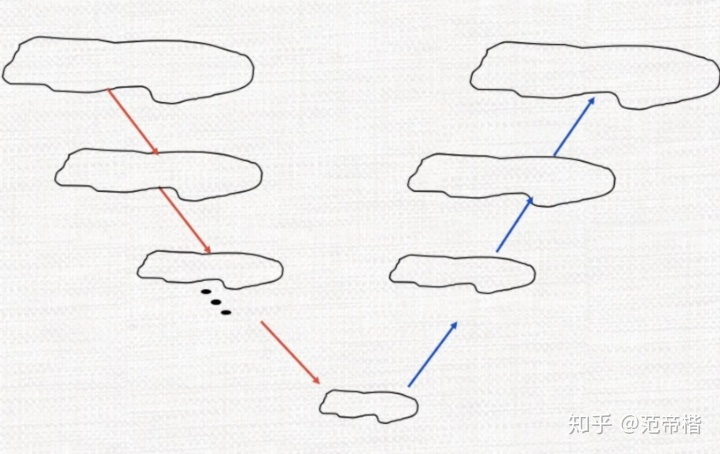这个方法实际上是比较简单的，网格如下所示：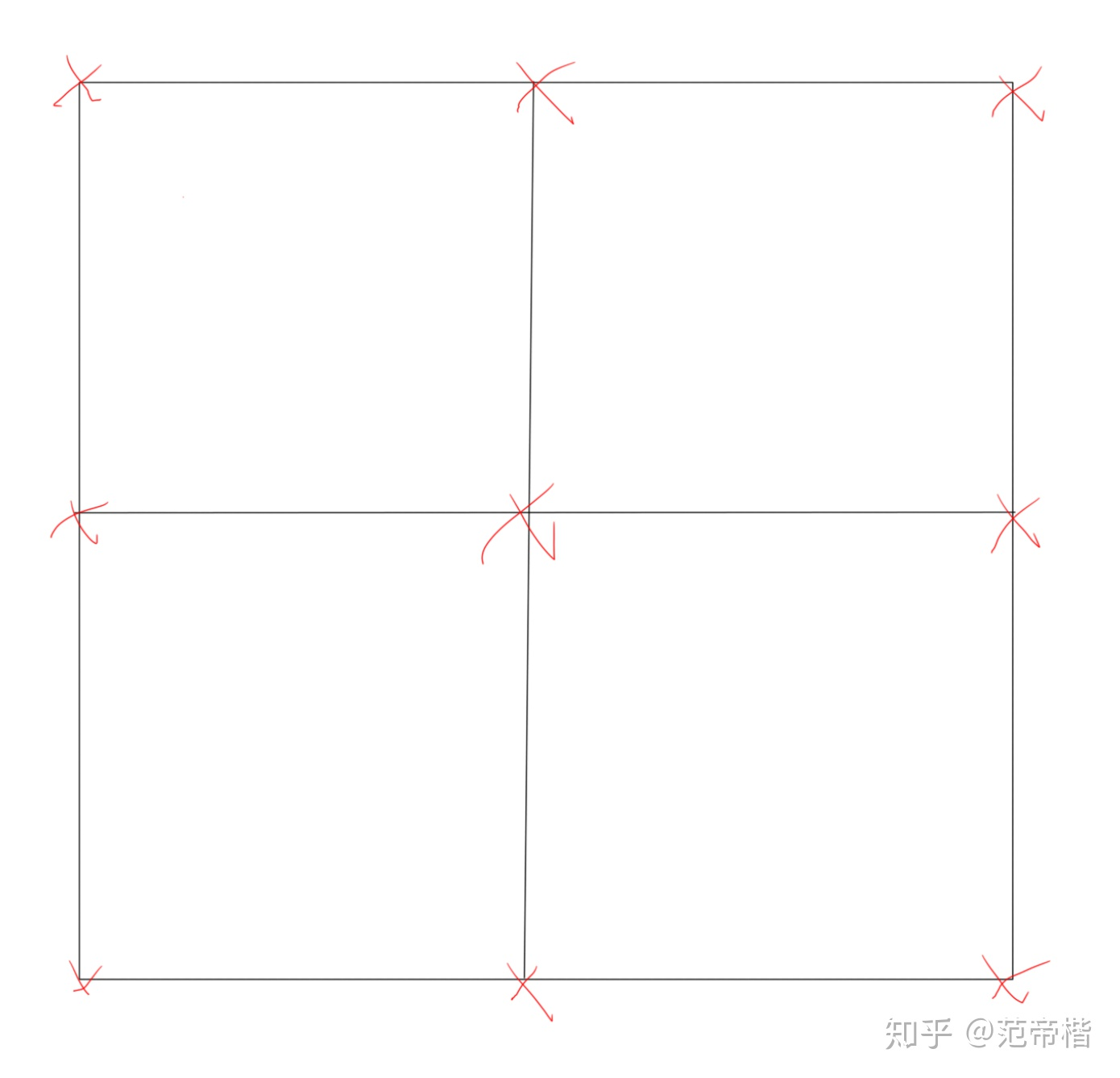红色的点代表粗网格上的节点，如果我们将网格细化，那么，可以得到细网格的点：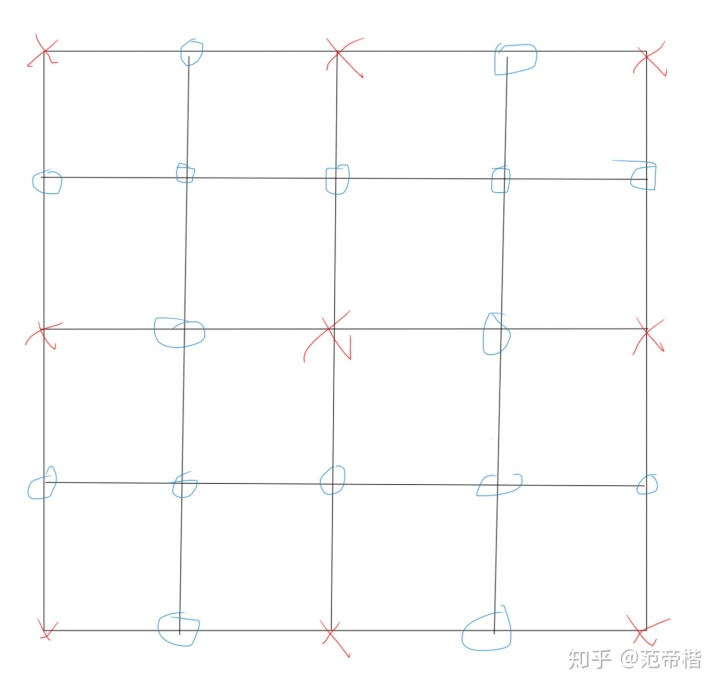这些点的值通过粗网格插值而来，也就是说，几何多重网格实际上是依赖于实际的网格，人为设定了粗网格和细网格，从而设置的一种多重网格算法。注意到这种方法细网格上的节点上一定有粗网格上的节点，因此原来如果粗细网格原来是同一个点，只需要将粗网格上的点赋值到细网格上，如果是新的点，那么需要对它进行插值，假设这个矩阵为，表示第k层网格上的节点，那么，因此我们只需要求解线性方程组：之后插值即可。但实际上这样做是不对的。回到最初的问题，我们要消除的是低频误差，也就是“光滑”误差，在粗网格上迭代后的结果实际上和光滑没有太多关系，我们迭代后的结果，实际上是消除了高频的误差，也就是将误差变得光滑了。 因此，我们将误差传到粗网格上实际上是更为合理的，因此我们最终的迭代思路实际上是不断修正误差，即：其中代表每层网格给出的插值后的补偿误差。 那么，我们的误差方程应该如何建立呢？ 定义残差:由此，我们有误差方程：由于我们希望将低频误差放到粗网格上进行消除，因此我们实际上将“限制”到了粗网格上计算，然后再插值回粗网格上进行“误差补偿”。 最终，我们实际上计算了：，然后插值得到，最后补偿到上。代数多重网格是否有一种方法可以依赖于系数矩阵本身的特性，从而设计一种多重网格方法，与实际网格无关，从而得到更好的收敛性呢？实际上是有的，这个方法就是代数多重网格。 由于我们现在没有了实际网格，那么实际上为了使用多重网格技术，我们需要回答两个问题：要选择哪些节点为粗网格上的节点如何插值对于问题1，实际上我们是需要准从两个原则：得到的粗网格节点能够通过插值表示细网格节点，或者说粗网格节点一定原来有和细网格节点相连。另一方面，我们希望粗节点能够尽量少，从而求解线性方程组的规模可以更小。 对于这个问题有个经典方法: 即Ruge提出来的方法对于问题2，回答了两个子问题： 1) 插值公式长什么样 2)粗网格上的线性方程组长什么样 通常如果插值矩阵为，那么粗网格上的线性方程组的系数矩阵构建为:因此实际上核心还是在于如何插值。假设表示连接i和j节点的边的权重，同时我们认为残差几乎为0，由此可以得到:由此:由于这些边连接的节点可以分为3类：细网格上的节点粗网格上的节点连接极弱的节点若用C表示粗节点误差集合，F表示细节点误差集合，W表示弱连接集合，可以将求和部分分为三块：由于弱连接集合的连接已经足够弱，因此我们强制认为这部分的,由此可以得到新的近似公式：对于粗网格上的$e_j$实际上在粗网格上已经算出，而细网格上的实际上是没有的。 对于这部分有连接的细网格上的，我们找到和它相连的所有粗网格的节点，通过公式：对它进行近似计算。
展开全文• 雅可比迭代法MATLAB程序：Function[x,k,index]=Jacobi(A,b,ep,it-max)%求线性方程组的雅可比法;%A为方程组的系数矩阵;%b为方程组的右端项;%x为方程组的解;%ep为精度要求,缺省值为le-5;%it_max为最大迭代次数,缺省...
雅可比迭代法的MATLAB程序：Function[x,k,index]=Jacobi(A,b,ep,it-max)%求线性方程组的雅可比法;% A为方程组的系数矩阵;% b为方程组的右端项;% x为方程组的解;% ep为精度要求,缺省值为le-5;% it_max为最大迭代次数,缺省值为100;% k为迭代次数;% index为指标变量,index=0表示计算失败,index=1表示计算成功;if nargin<4it_max=100;endif nargin<3ep=le-5;endn=length(A);k=0;x=zeros(n,1);y=zeros(n,1);index=1;while k<=it_maxfor i=1:nif abs (A(i,i))index=0;return;endy(i)=(b(i)-A(i,1:n)*x(1:n)+A(i,i)*x(i))/A(i,i);endif norm(y-x,inf)break;endk=k+1;x=y;end高斯-赛德尔迭代的MATLAB程序Function[x,k,index]=Gau-seidel(A,b,ep,it-max)%求线性方程组的高斯-赛德尔迭代法;% A为方程组的系数矩阵;% b为方程组的右端项;% x为方程组的解;% ep为精度要求,缺省值为le-5;% it_max为最大迭代次数,缺省值为100;% k为迭代次数;% index为指标变量,index=0表示计算失败,index=1表示计算成功;if nargin<4it_max=100;
展开全文• 传统迭代法中的雅可比迭代法，算法原理和matlab程序代码全部包含，还有一个算例
• 1.雅可比迭代法：function [x,n]=jaccbi(A,b,x0,eps,t) if nargin==3;eps=1e-6;m=200;elseif nargin<3error('输入的数有误'); return;elseif nargin==5m=t;endD=diag(diag(A));L=-tril(A,-1);U=-triu(A,1);B=D\(L...
1.雅可比迭代法：function [x,n]=jaccbi(A,b,x0,eps,t) if nargin==3;eps=1e-6;m=200;elseif nargin<3error('输入的数有误');     return;elseif nargin==5m=t;endD=diag(diag(A));L=-tril(A,-1);U=-triu(A,1);B=D\(L+U);f=D\b;x=B*x0+f;n=1;while norm(x-x0)>=epsx0=x;x=B*x0+f;n=n+1;if(n>=m)disp('可能不收敛');         return;end;end2.高斯—赛德尔迭代法： function [x,n]=gsdddy(A,b,x0,eps,t) if nargin==3;eps=1e-6;m=200;elseif nargin<3error('输入有误');return;elseif nargin==5m=t;endD=diag(diag(A));L=-tril(A,-1);U=-triu(A,1);B=(D-L)\U;f=(D-L)\b;x=B*x0+f;n=1;while norm(x-x0)>=epsx0=x;x=B*x0+f;
展开全文• function [y,n]=jacobi(A,b,x0,ep) if nargin==3 ep=1.0e-6; else if nargin(diag(A; L=-tril(A,-1; U=-triu(A,10; B=D\(L+U; f=D\b; y=B*x0+f; n=1; while norm(y-x0>=ep x0=y; y=
• function [y,n]=jacobi(A,b,x0,ep) if nargin==3 ep=1.0e-6; else if nargin(diag(A; L=-tril(A,-1; U=-triu(A,10; B=D\(L+U; f=D\b; y=B*x0+f; n=1; while norm(y-x0>=ep x0=y; y=B
• 文章目录前言1 直接法2 迭代法小结 前言 今天我们要说的就是数值微积分，赶紧看看他和高等数学中的微积分有什么区别吧。本文是科学计算与MATLAB语言专题六第2小节的学习笔记，如果大家有时间的话，可以去听听课，...算法 线性代数
• 于是 可以写成 即是 下面我们写一个代码，来程序实现一下雅可比迭代方法：% 雅可比迭代法，计算线性方程组的解 下面我们以一个方程组为例来测试该代码：在编辑界面输入如下命令：a 最后得出如下结果：...
• 代码块1：雅可比迭代法： clear; clc; fprintf('雅可比迭代法解线性方程组:\n') n=input('请输入系数矩阵A的阶数:n='); A=input('请输入系数矩阵A:A='); b1=input('请输入结果向量b1:b1=');%b为行向量，计算时需要...算法 线性代数 矩阵
• 利用牛顿-雅可比迭代法求非线性方程组Ax=b的一个根,压缩包里包含了求解非线性方程组的代码，只要用MATLAB软件打开程序运行即可
• function root= Secant( f,a,b,eps ) if(nargin==3) eps=1.0e-4; end f1=subs(sym(f),findsym(sym(f)),a); f2=subs(sym(f),findsym(sym(f)),b); if(f1==0) root=a; end if(f2==0) ...if(f1*f2&...
• A=[4,3,0;3,4,-1;0,-1,4]; b=[24,30,-24]; x=jokebi(A,b); function x2=jokebi(B,c) D=diag(diag(B)); U=D-triu(B); L=D-(triu(B))'; x1=(rand(1,size(B,1)))';... x2=inv(D)*(U+L)*x1+inv(D
• 题目一： 1.假设理想状态下的弹道轨迹满足抛物线方程y=a+bx+cx^2（注：真实状态下的轨迹并不是那样，一般发射后段的轨迹比较倾斜，这是因为后段受空气阻力的影响比较大。...3.matlab代码： A=[1,1,1...数值分析
• function [X_reality,n_reality] = ...% A为迭代的系数矩阵 % b为方程组右边的常数项（列向量） % X_start为迭代的初始向量 % n_limit为最大允许迭代的次数 % tolerance为精度上限值 %% % X_reality为最后结果...
• 雅可比迭代，二分法，增值寻根，高斯解方程组的MATLAB源码,课堂作业分享，运行是没有问题的，但是效率不一定高。希望能够互相学习~
• 但对于A 的阶数n很大，零元素较多的大型稀疏矩阵方程组，利用迭代法求解则更为合适。迭代法通常适用于A中有大量零元素的特点。 简单迭代法是究竟是什么呢？给下面这个例子你就懂了 E.G.已知9x2=sinx+19x^2 = sinx+...jacobi 线性方程组
• 直接法：一般实和求解低阶稠密线性方程组。 迭代法：适用于求解大型稀疏矩阵线性方程组；要考虑算法的收敛性
• 雅可比和SOR超松弛迭代法matlab程序.rar
• 雅可比（Jacobi）迭代法解线性方程组的Matlab实现 迭代法解线性方程组的基本思想是构造一串收敛到解的序列，即建立一种从已有近似解计算新的近似解的规则，有不同的计算规则得到不同的迭代法。 本文是常用的迭代法之...算法
• 本节，我们介绍超松弛迭代法。下面我们对该算法进行收敛性分析：这里我们解释一下什么叫严格对角占优矩阵：严格对角占优矩阵就是矩阵主对角线元素的的模大于与他同行的其他元素的模的总和。下面我们具体代码实现一下...
• 数值分析实验内容，用matlab写程序实现雅可比Jacobi迭代法迭代法解线性方程组的基本思想是构造一串收敛到解的序列，即建立一种从已有近似解计算新的近似解的规则，有不同的计算规则得到不同的迭代法
• 线性方程组迭代解法——雅可比（Jacobi）迭代...%%%采用雅可比迭代法求解方程组的数值解； %%%输出数值解x。 [~,m]=size(A); A_diag=diag(A); A(1:m+1:end)=0; flag=1; x=x0; while flag for i=1:m x(i)=(b(i)-A(i,:)算法
•  %B=I-D^(-1)*A ,B是迭代用的矩阵 g=Dn*b; %g=D^(-1)*b ,是迭代用的向量 x=ones(size(A,1),1); %让初始x值为 [1 1 1]' %初始值随意给3个值就可以，这里简单给三个1 TF=1;tol=1e-7; %设定迭代循环标志TF和...
• clc clear n = input('请输入矩阵阶数：\n'); for i = 1:n fprintf('请输入矩阵第%d行\n',i); A(i,:) = input('');...B(:,1) = input('请输入B向量:\n');... fprintf('第%d次迭代结果：\n',i); x2 end# matlab雅可比迭代法matlab 订阅